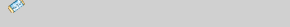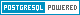토픽을 선택하세요 DBMS MySQL PostgreSQL Firebird Oracle Informix Sybase MS-SQL DB2 Cache CUBRID LDAP ALTIBASE Tibero 공지사항 자유게시판 구인|구직 운영게시판
 UserID Passwd
Database
DBMS
MySQL
PostgreSQL
Firebird
Oracle
Informix
Sybase
MS-SQL
ㆍDB2
Cache
CUBRID
LDAP
ALTIBASE
Tibero
DB 문서들
스터디
Community
공지사항
자유게시판
구인|구직
DSN 갤러리
도움주신분들
운영게시판
최근게시물
 DB2 Q&A 1705 게시물 읽기 News | Q&A | Columns | Tutorials | Devel | Files | Links
 No. 1705
작성자 작성일 조회수 db2 sql system error. 지창용(fever1020) 2008-08-18 18:34 12,062
 update payroll.pbpaymst_jj b set b.s11000 = ( (case       when b.px7000 = 1       then ( select family1 from payroll.pwtaxtbl where appym = (select ifnull(max(appym), '200701') from payroll.pwtaxtbl) and framt < b.px3000 and toamt >= b.px3000)       when b.px7000 = 2       then ( select family2 from payroll.pwtaxtbl where appym = (select ifnull(max(appym), '200701') from payroll.pwtaxtbl) and framt < b.px3000 and toamt >= b.px3000)       when b.px7000 = 3 and b.px6000 > 1       then ( select family3b from payroll.pwtaxtbl where appym = (select ifnull(max(appym), '200701') from payroll.pwtaxtbl) and framt < b.px3000 and toamt >= b.px3000)       when b.px7000 = 3 and b.px6000 < 2       then ( select family3 from payroll.pwtaxtbl where appym = (select ifnull(max(appym), '200701') from payroll.pwtaxtbl) and framt < b.px3000 and toamt >= b.px3000)       when b.px7000 = 4 and b.px6000 > 1       then ( select family4b from payroll.pwtaxtbl where appym = (select ifnull(max(appym), '200701') from payroll.pwtaxtbl) and framt < b.px3000 and toamt >= b.px3000)       when b.px7000 = 4 and b.px6000 < 2       then ( select family4 from payroll.pwtaxtbl where appym = (select ifnull(max(appym), '200701') from payroll.pwtaxtbl) and framt < b.px3000 and toamt >= b.px3000)       when b.px7000 = 5 and b.px6000 > 1       then ( select family5b from payroll.pwtaxtbl where appym = (select ifnull(max(appym), '200701') from payroll.pwtaxtbl) and framt < b.px3000 and toamt >= b.px3000)       when b.px7000 = 5 and b.px6000 < 2       then ( select family5 from payroll.pwtaxtbl where appym = (select ifnull(max(appym), '200701') from payroll.pwtaxtbl) and framt < b.px3000 and toamt >= b.px3000)       when b.px7000 = 6 and b.px6000 > 1       then ( select family6b from payroll.pwtaxtbl where appym = (select ifnull(max(appym), '200701') from payroll.pwtaxtbl) and framt < b.px3000 and toamt >= b.px3000)       when b.px7000 = 6 and b.px6000 < 2       then ( select family6 from payroll.pwtaxtbl where appym = (select ifnull(max(appym), '200701') from payroll.pwtaxtbl) and framt < b.px3000 and toamt >= b.px3000)       when b.px7000 = 7 and b.px6000 > 1       then ( select family7b from payroll.pwtaxtbl where appym = (select ifnull(max(appym), '200701') from payroll.pwtaxtbl) and framt < b.px3000 and toamt >= b.px3000)       when b.px7000 = 7 and b.px6000 < 2       then ( select family7 from payroll.pwtaxtbl where appym = (select ifnull(max(appym), '200701') from payroll.pwtaxtbl) and framt < b.px3000 and toamt >= b.px3000)       when b.px7000 = 8 and b.px6000 > 1       then ( select family8b from payroll.pwtaxtbl where appym = (select ifnull(max(appym), '200701') from payroll.pwtaxtbl) and framt < b.px3000 and toamt >= b.px3000)       when b.px7000 = 8 and b.px6000 < 2       then ( select family8 from payroll.pwtaxtbl where appym = (select ifnull(max(appym), '200701') from payroll.pwtaxtbl) and framt < b.px3000 and toamt >= b.px3000)       when b.px7000 = 9 and b.px6000 > 1       then ( select family9b from payroll.pwtaxtbl where appym = (select ifnull(max(appym), '200701') from payroll.pwtaxtbl) and framt < b.px3000 and toamt >= b.px3000)       when b.px7000 = 9 and b.px6000 < 2       then ( select family9 from payroll.pwtaxtbl where appym = (select ifnull(max(appym), '200701') from payroll.pwtaxtbl) and framt < b.px3000 and toamt >= b.px3000)       when b.px7000 = 10 and b.px6000 > 1       then ( select family10b from payroll.pwtaxtbl where appym = (select ifnull(max(appym), '200701') from payroll.pwtaxtbl) and framt < b.px3000 and toamt >= b.px3000)       when b.px7000 = 10 and b.px6000 < 2       then ( select family10 from payroll.pwtaxtbl where appym = (select ifnull(max(appym), '200701') from payroll.pwtaxtbl) and framt < b.px3000 and toamt >= b.px3000)       when b.px7000 = 11 and b.px6000 > 1       then ( select family11b from payroll.pwtaxtbl where appym = (select ifnull(max(appym), '200701') from payroll.pwtaxtbl) and framt < b.px3000 and toamt >= b.px3000)       when b.px7000 = 11 and b.px6000 < 2       then ( select family11 from payroll.pwtaxtbl where appym = (select ifnull(max(appym), '200701') from payroll.pwtaxtbl) and framt < b.px3000 and toamt >= b.px3000)       when b.px7000 > 11 and b.px6000 > 1       then ( select family3b from payroll.pwtaxtbl where appym = (select ifnull(max(appym), '200701') from payroll.pwtaxtbl) and framt < b.px3000 and toamt >= b.px3000)       when b.px7000 > 11 and b.px6000 < 2       then ( select family3 from payroll.pwtaxtbl where appym = (select ifnull(max(appym), '200701') from payroll.pwtaxtbl) and framt < b.px3000 and toamt >= b.px3000)       else '1' end) )  where b.appdt = '200808'  and   b.paydiv = 'S'  and   b.px3000 >= 800000 조건이 좀복잡해서 이런식으로 쿼리를 만들엇는데요 winsql에서 아래와같은 에러를 뱉어내는데요.... Error: SQL0901 - SQL system error. (State:S1000, Native Code: FFFFFC7B) 원인이 무엇인지...ㅠㅠ 이거때문에 지금 1주일째고생하고있음..ㅠㅠ 살려주십시오...
이 글에 대한 댓글이 총 3건 있습니다.

1. federation을 사용하는것 같은데 link가 걸린 테이블 목록을 알 수 있을까요?
2. 문장이 길어지면서 발생하는 것이 아닌가 하는 의문이 드는데요 case 문을 하나만 돌릴 경우에도 문제가 발생하나요?

db2 "? SQL0901"

SQL0901N 심각하지 않은 시스템 오류로 인해 SQL문이 실패했습니다.
후속 SQL문은 처리할 수 있습니다. (이유 "<reason>".)

설명:

시스템 오류로 인해 오류가 발생했습니다. SQL문 처리가 이유
"<reason>"(이것은 영어에만 해당되고 IBM 지원 담당자에게만
유용함)(으)로 종료되었습니다.

사용자 조치:

메시지 번호(SQLCODE)와 이유 "<reason>"을(를) 기록해 두십시오.

운영 체제 명령 프롬프트에서 ITF(Independent Trace Facility)를
호출하십시오.  그런 다음, 다음 정보를 가지고 기술 서비스 담당
부서에게 문의하십시오.

o   문제점 설명

o   SQLCODE

o   이유 "<reason>"

o   가능한 경우, SQLCA 내용

o   가능한 경우, 추적 파일

페더레이티드 시스템 사용자: 요청에 실패한 데이터 소스에 대해
문제점을 분리하고(SQL문 처리에 실패한 데이터 소스를 판별하려면
문제점 판별 안내서 참조) 해당 데이터 소스에 대해 필요한 진단
단계를 수행하십시오. 데이터 소스에 대한 문제점 판별 프로시저는
다양하므로, 적용되는 데이터 소스 매뉴얼을 참조하십시오.

sqlcode :  -901

sqlstate : 58004

조는 냥이님이 2008-08-19 08:52에 작성한 댓글입니다. Edit

자체 해결했습니다....

제가 만든 쿼리문이 아니라 원래 만들어져잇던 쿼리라 잘될거라 예상햇는데

이런에러가 나와서 황당햇었습니다..

set에 대입되는 괄호부분을 쿼리로 만들고 from절에 then절의 서브쿼리를 하나 붙여주었더니

잘되는군요.....federation을 사용하는것같다고하시는데

1.federation이 무슨말인지 ㅋㅋ..;;초짜라 지송...

2. 문장길어져서 나오는 문제면 힙이 모자르다고 나오는거아니었나요?

시스템에러는 첨봐서...ㅋㅋㅋ;;

지창용(fever1020)님이 2008-08-19 16:50에 작성한 댓글입니다.

문장이 길어지면 힙부족 메시지가 떨어집니다. ㅎㅎ
제 의도는 비슷한 sql이 반복되길래 줄이면 해결이 될수도 있을것 같아 말씀드린 겁니다.

조는 냥이님이 2008-08-19 17:32에 작성한 댓글입니다.
이 댓글은 2008-08-19 17:33에 마지막으로 수정되었습니다. Edit
[Top]
No.
제목
작성자
작성일
조회
1709인덱스 스크립트 
이도희
2008-08-22
9553
1707마지막 특정문자 이후로 자르는 법 문의드립니다. 
궁금이
2008-08-22
10455
1706테이블 RENAME시 발생되는 문제에 대해 다시 질문 드립니다. 
이도희
2008-08-19
10360
1705db2 sql system error. 
지창용
2008-08-18
12062
1704OS/390 DB2 5.1 JDBC 문의드립니다.
김황만
2008-08-18
9190
1703다중 데이터베이스 파티션 질문 
이도희
2008-08-14
10360
1702codepage 질문 
이도희
2008-08-11
10908
작업시간: 0.058초,